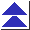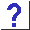# 4. Pointers

4.1 What are pointers really good for, anyway?

4.2 I'm trying to declare a pointer and allocate some space for it, but it's not working. What's wrong with this code?

```char *p;
*p = malloc(10);```

4.3 Does *p++ increment p, or what it points to?

4.4 I'm trying to use pointers to manipulate an array of ints. What's wrong with this code?

```	int array, i, *ip;
for(i = 0; i < 5; i++) array[i] = i;
ip = array;
printf("%d\n", *(ip + 3 * sizeof(int)));
```
I expected the last line to print 3, but it printed garbage.

4.5 I have a char * pointer that happens to point to some ints, and I want to step it over them. Why doesn't

`((int *)p)++;`
work?

4.6 Why can't I perform arithmetic on a void * pointer?

4.7 I've got some code that's trying to unpack external structures, but it's crashing with a message about an ``unaligned access.'' What does this mean?

4.8 I have a function which accepts, and is supposed to initialize, a pointer:

```	void f(int *ip)
{
static int dummy = 5;
ip = &dummy;
}
```
But when I call it like this:
```	int *ip;
f(ip);
```
the pointer in the caller remains unchanged.

4.9 Suppose I want to write a function that takes a generic pointer as an argument and I want to simulate passing it by reference. Can I give the formal parameter type void **, and do something like this?

```	void f(void **);
double *dp;
f((void **)&dp);
```

4.10 I have a function

```	extern int f(int *);
```
which accepts a pointer to an int. How can I pass a constant by reference? A call like
```	f(&5);
```
doesn't seem to work.

4.11 Does C even have ``pass by reference''?

4.12 I've seen different syntax used for calling functions via pointers. What's the story?

4.13 What's the total generic pointer type? My compiler complained when I tried to stuff function pointers into a void *.

4.14 How are integers converted to and from pointers? Can I temporarily stuff an integer into a pointer, or vice versa?

4.15 How do I convert an int to a char *? I tried a cast, but it's not working.

4.16 What's wrong with this declaration?

`char* p1, p2;`
I get errors when I try to use p2.

4.17 What are ``near'' and ``far'' pointers?